## Holiday Fun Bundle!

 table.module-2{width:72.45%;padding:0}table div table+table+table div table{width:72.45%;float:none;margin-left:auto;margin-right:auto;padding:0}table div table+table+table div table a{border:0 none;text-decoration:none}table div table+table+table div table img{width:100%!important;border:0 none;text-decoration:none}table div table+table+table div table td{width:100%;padding:0}/* styles */
 table.module-3{width:75.66%;padding:0}table div table+table+table+table div table{width:75.66%;float:none;margin-left:auto;margin-right:auto;padding:0}table div table+table+table+table div table a{border:0 none;text-decoration:none}table div table+table+table+table div table img{width:100%!important;border:0 none;text-decoration:none}table div table+table+table+table div table td{width:100%;padding:0}/* styles */
 table.module-4{width:75.09%;padding:0}table div table+table+table+table+table div table{width:75.09%;float:none;margin-left:auto;margin-right:auto;padding:0}table div table+table+table+table+table div table a{border:0 none;text-decoration:none}table div table+table+table+table+table div table img{width:100%!important;border:0 none;text-decoration:none}table div table+table+table+table+table div table td{width:100%;padding:0}/* styles */

# Holiday Fun Bundle 21!

4 projects designed by Karen Montgomery and access to a private Facebook group where Karen will be posting video classes!

The feature project is a table runner made from a panel - we have 3 choices. You will never look at a panel the same way again after Karen's class!

The bundle also includes kits for 2 placemats, a wine butler and your choice of fabrics for a Travel Tote!

Holiday Fun Bundles include patterns, fabric and all the "stuff" needed to complete the 4 projects.
Kits will ship starting October 15, class starts November 6 and will be available until the end of the year.

 table div table+table+table+table+table+table+table+table div table{width:100%;padding:0}table div table+table+table+table+table+table+table+table div table img{width:96.23%;padding:0;float:none}table div table+table+table+table+table+table+table+table div table td{width:100%;padding:0 1.88% 18px}/* styles */# It's Back to Basics this Week!

Great basics in all the colors you use! You can't go wrong putting any of these in your next project or your stash!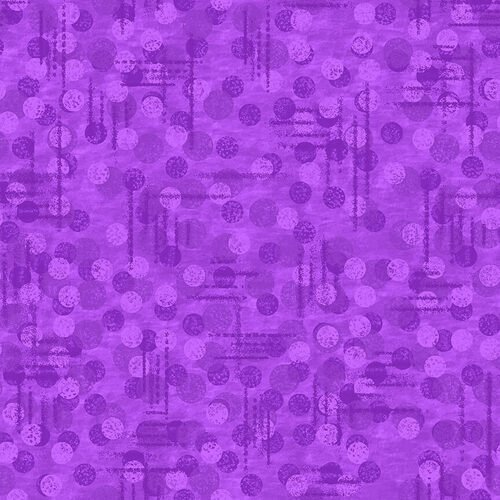# Jot Dot!

Jot Dot quickly became a favorite and you can see why!

It has some texture and depth without stealing the show!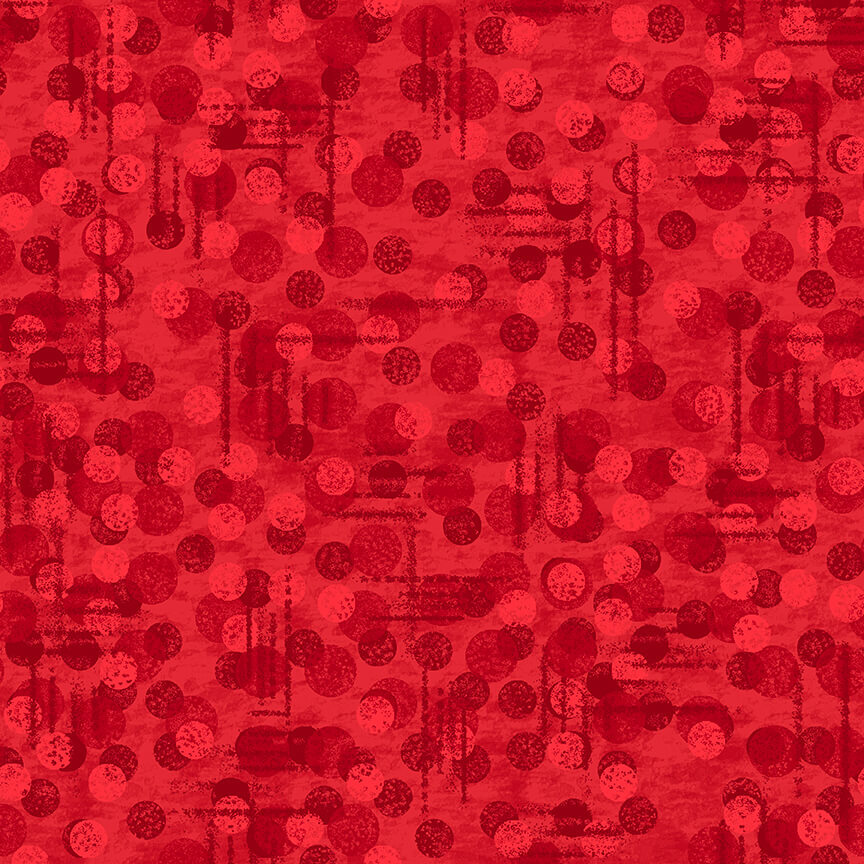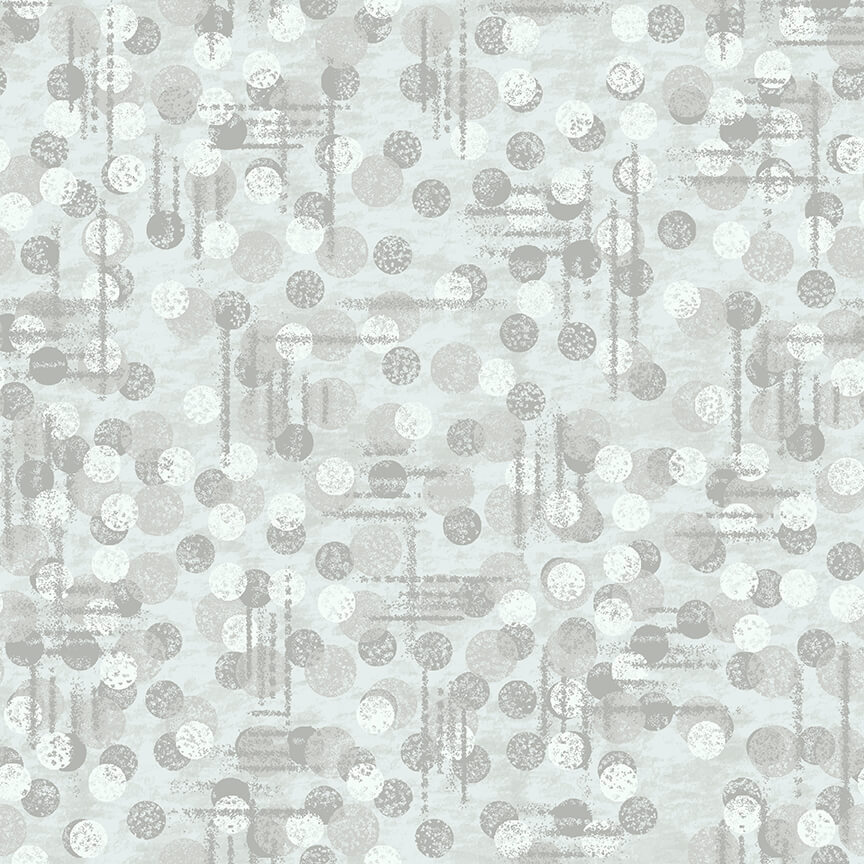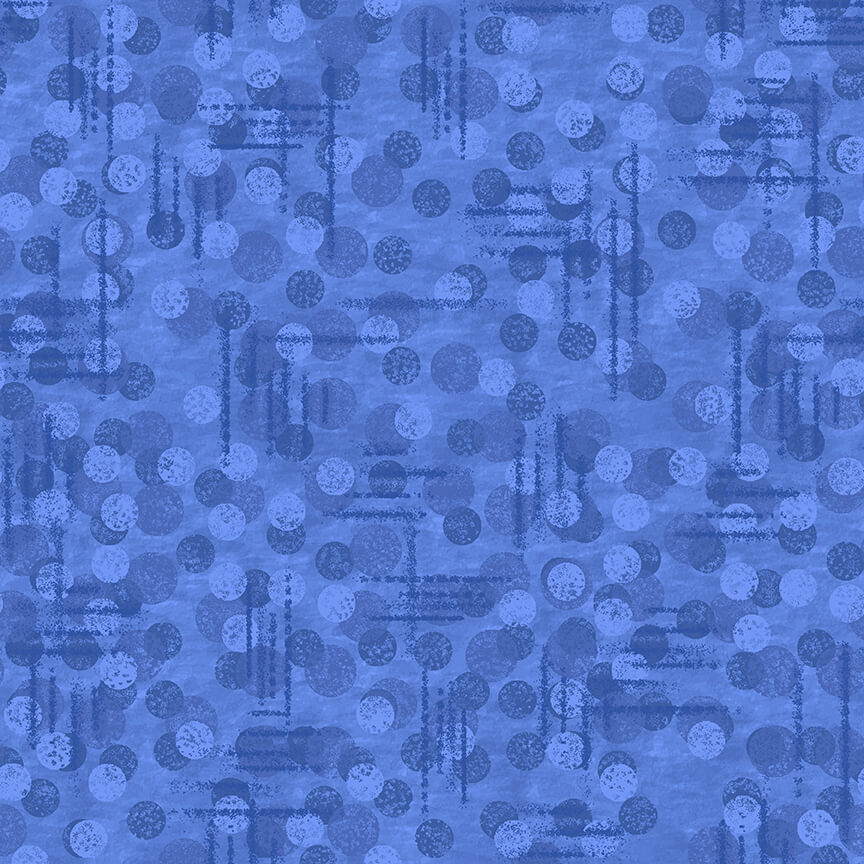table div table+table+table+table+table+table+table+table+table+table+table+table+table div table{width:100%;padding:0}table div table+table+table+table+table+table+table+table+table+table+table+table+table div table img{width:96.23%;padding:0;float:none}table div table+table+table+table+table+table+table+table+table+table+table+table+table div table td{width:100%;padding:0 1.88% 18px}/* styles */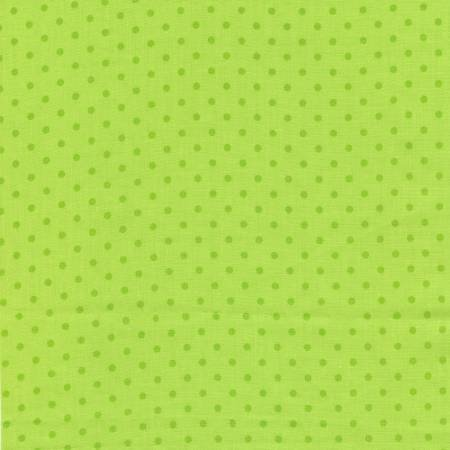# Everyone Loves Polka Dots!

Polka Dots add that "little something extra" and they are always fun!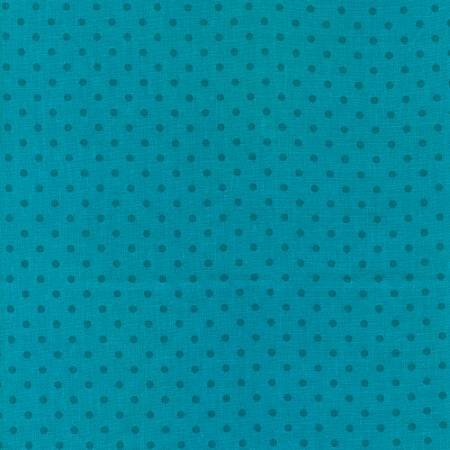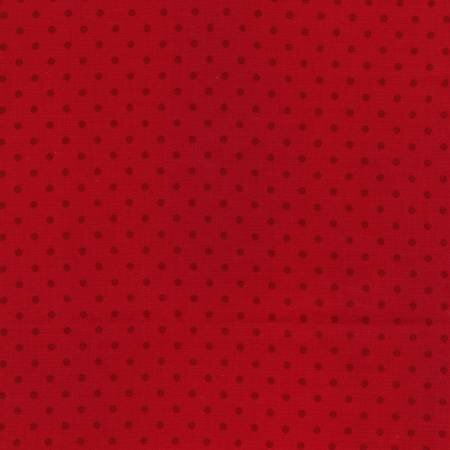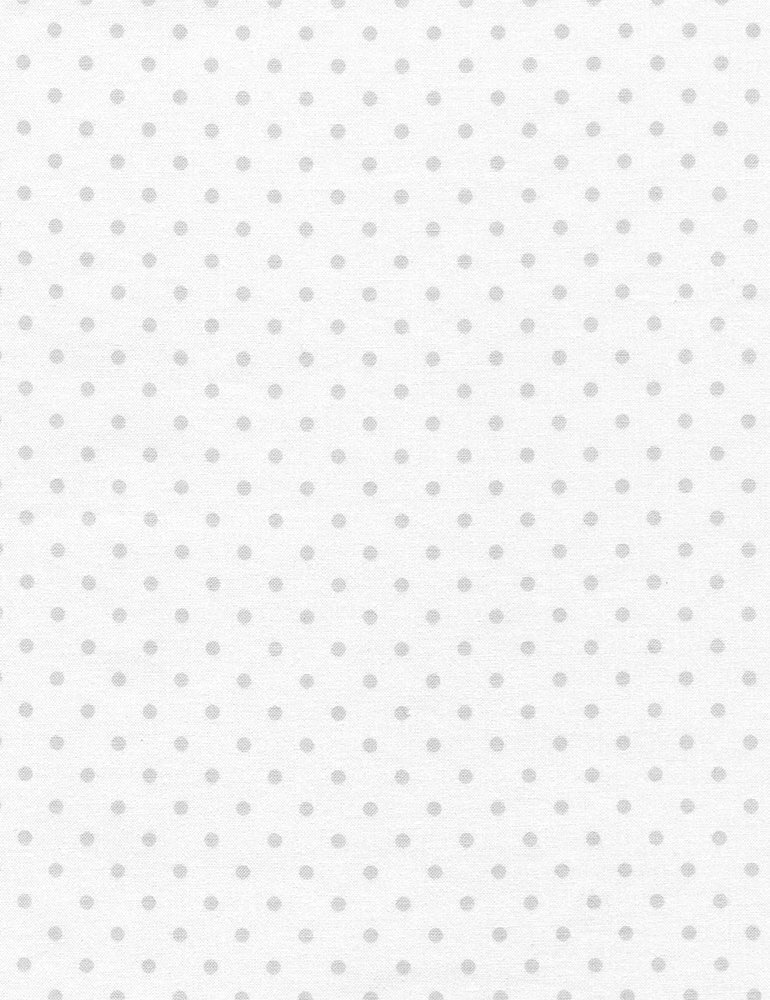table div table+table+table+table+table+table+table+table+table+table+table+table+table+table+table+table+table div table{width:100%;padding:0}table div table+table+table+table+table+table+table+table+table+table+table+table+table+table+table+table+table div table img{width:96.23%;padding:0;float:none}table div table+table+table+table+table+table+table+table+table+table+table+table+table+table+table+table+table div table td{width:100%;padding:0 1.88% 18px}/* styles */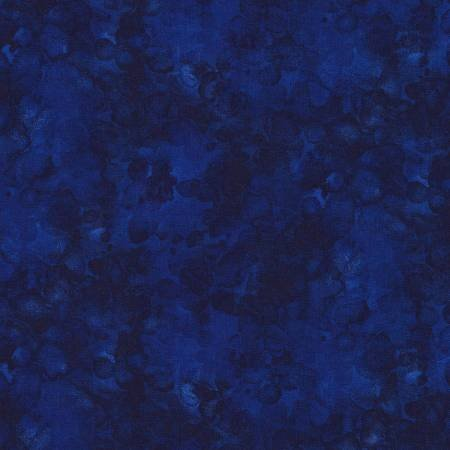# Solid-ish

Such a funny name for such a beautiful fabric! Solid-ish go especially well with florals, but they look great with nearly all fabrics - great stash builder!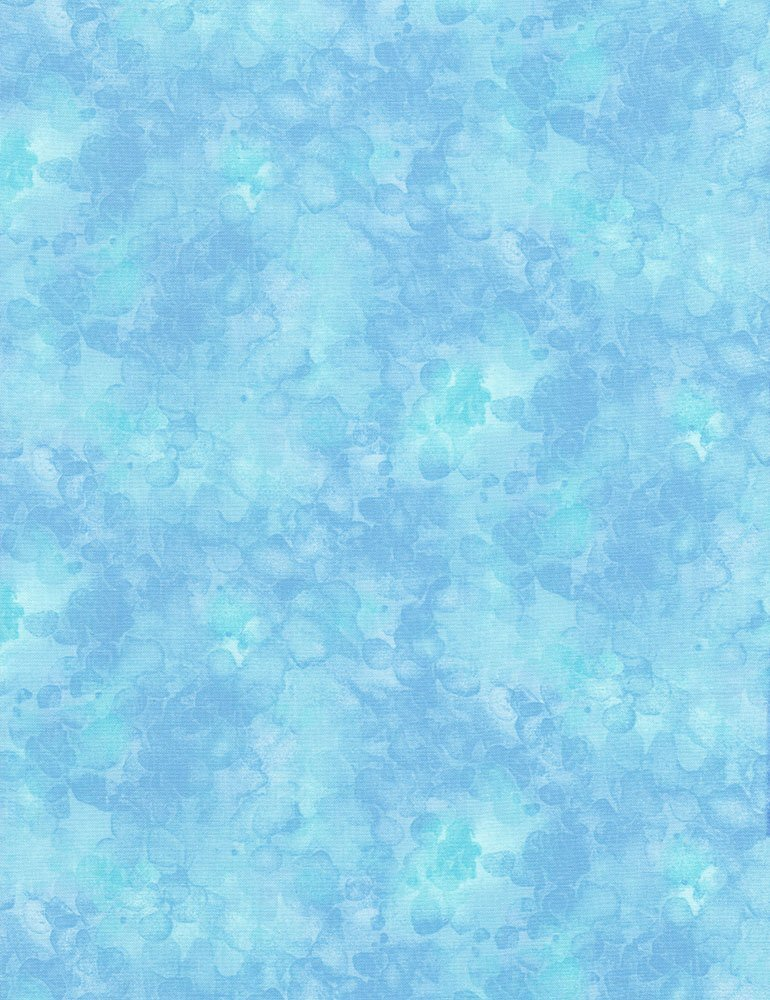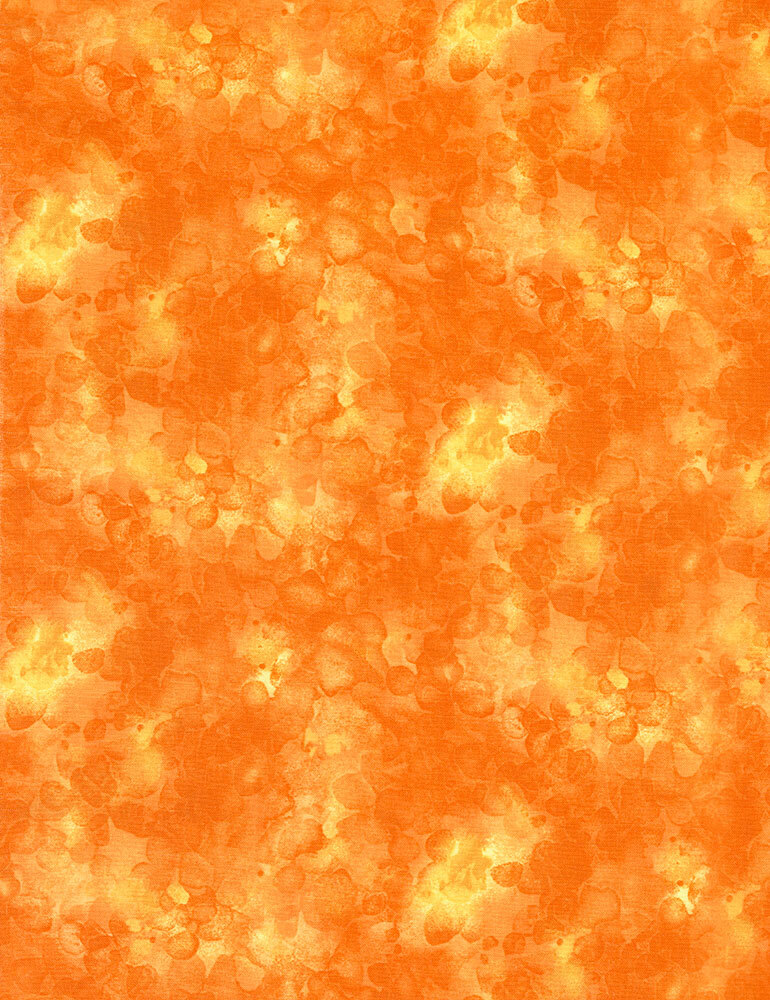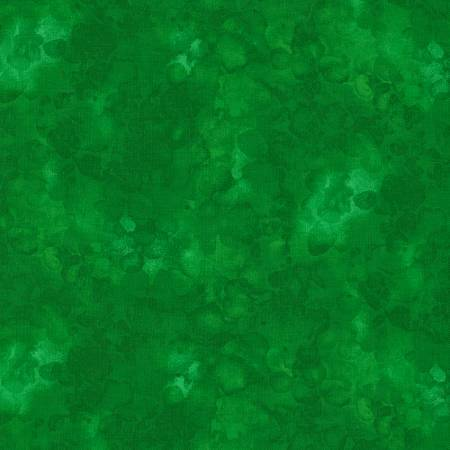table div table+table+table+table+table+table+table+table+table+table+table+table+table+table+table+table+table+table+table+table+table div table{width:100%;padding:0}table div table+table+table+table+table+table+table+table+table+table+table+table+table+table+table+table+table+table+table+table+table div table img{width:96.23%;padding:0;float:none}table div table+table+table+table+table+table+table+table+table+table+table+table+table+table+table+table+table+table+table+table+table div table td{width:100%;padding:0 1.88% 18px}/* styles */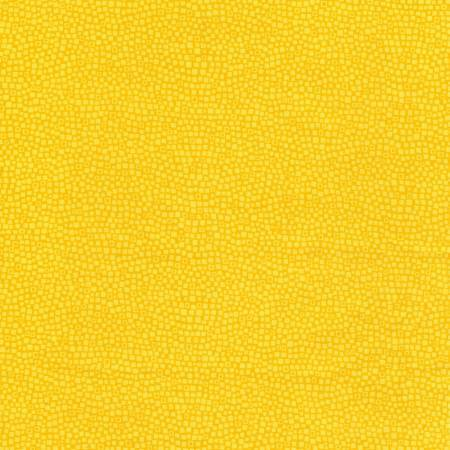# Blockbuster!

If you look closely, you will see tone on tone blocks in this blender!
I picked all your favorite colors for you!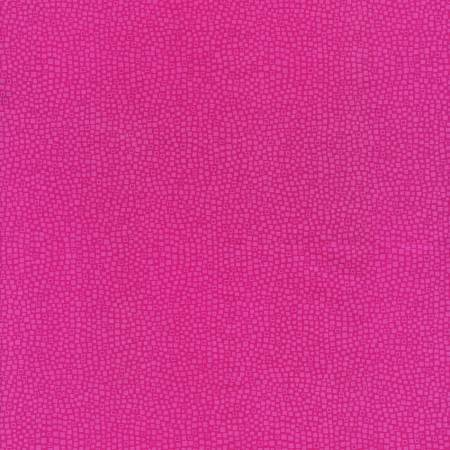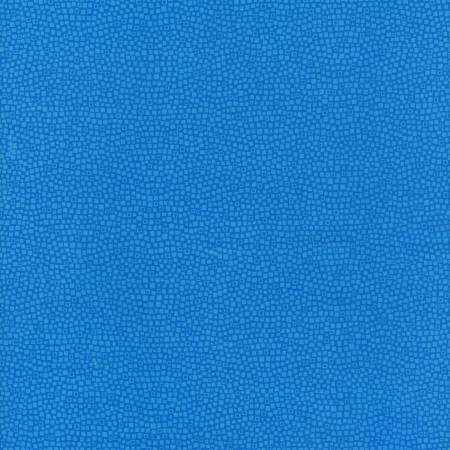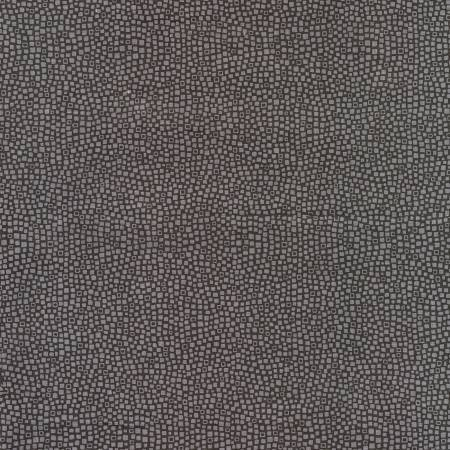table div table+table+table+table+table+table+table+table+table+table+table+table+table+table+table+table+table+table+table+table+table+table+table+table+table div table{width:100%;padding:0}table div table+table+table+table+table+table+table+table+table+table+table+table+table+table+table+table+table+table+table+table+table+table+table+table+table div table img{width:96.23%;padding:0;float:none}table div table+table+table+table+table+table+table+table+table+table+table+table+table+table+table+table+table+table+table+table+table+table+table+table+table div table td{width:100%;padding:0 1.88% 18px}/* styles */# Candy Corn Quilt Shoppe!

Available in a Sewing Version or a Machine Embroidery version, Candy Corn Quilt Shoppe is adorable!
Be sure to get the embellishment kit, it has all kinds of fun goodies: buttons, tulle, ribbon, glitter sheets and lots more!
We have a limited number of boxed fabric kits available - be sure to get yours!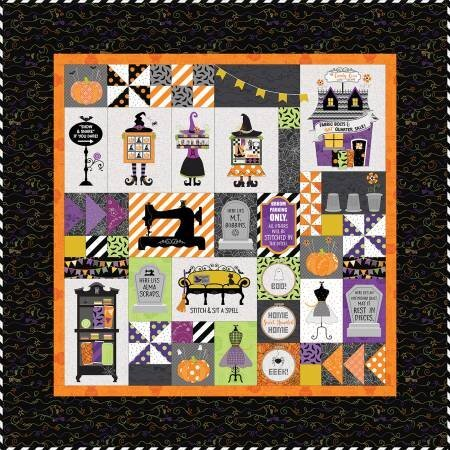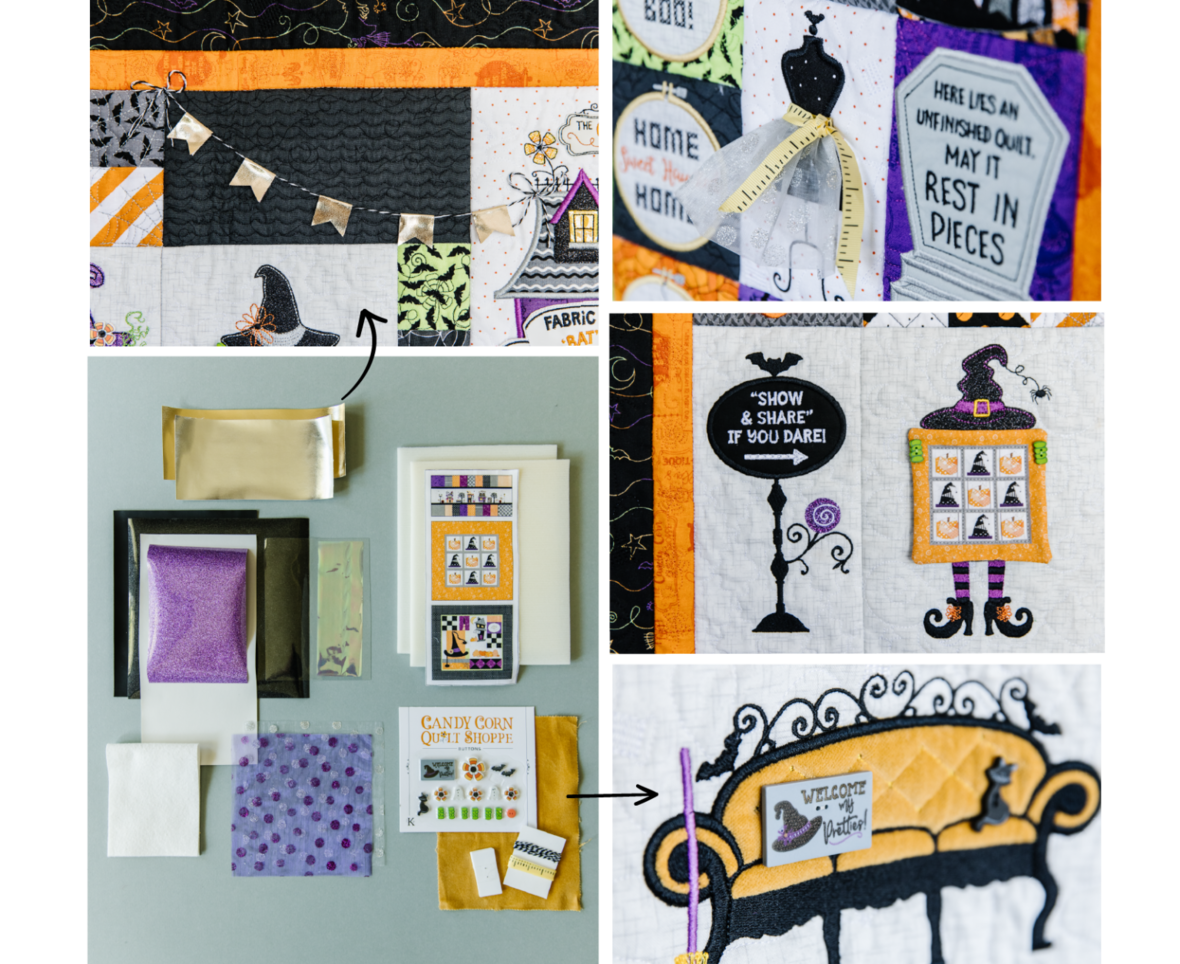table div table+table+table+table+table+table+table+table+table+table+table+table+table+table+table+table+table+table+table+table+table+table+table+table+table+table+table+table+table div table{width:100%;padding:0}table div table+table+table+table+table+table+table+table+table+table+table+table+table+table+table+table+table+table+table+table+table+table+table+table+table+table+table+table+table div table img{width:96.23%;padding:0;float:none}table div table+table+table+table+table+table+table+table+table+table+table+table+table+table+table+table+table+table+table+table+table+table+table+table+table+table+table+table+table div table td{width:100%;padding:0 1.88% 18px}/* styles *//* styles */ Sunday Soiree! FB Live is the place to be Sunday at 7pm! You will be the 1st to see new fabrics, notions, kits and other great things that you need to know about! We put together fun kits that often sell out that very night - you don't want to miss out! There will be prizes, live demos and special pricing! You will be able to purchase during the Live Broadcast and we can ship - sort of like Stuff Your Stocking but all in one night. If you miss the FB Live - not to worry! You can view the replay on our FB Page AND you can still purchase at the special prices until 12pm Monday! Join me for a fun evening!
 table div table+table+table+table+table+table+table+table+table+table+table+table+table+table+table+table+table+table+table+table+table+table+table+table+table+table+table+table+table+table+table+table+table div table{width:100%;padding:0}table div table+table+table+table+table+table+table+table+table+table+table+table+table+table+table+table+table+table+table+table+table+table+table+table+table+table+table+table+table+table+table+table+table div table img{width:96.23%;padding:0;float:none}table div table+table+table+table+table+table+table+table+table+table+table+table+table+table+table+table+table+table+table+table+table+table+table+table+table+table+table+table+table+table+table+table+table div table td{width:100%;padding:0 1.88% 18px}/* styles */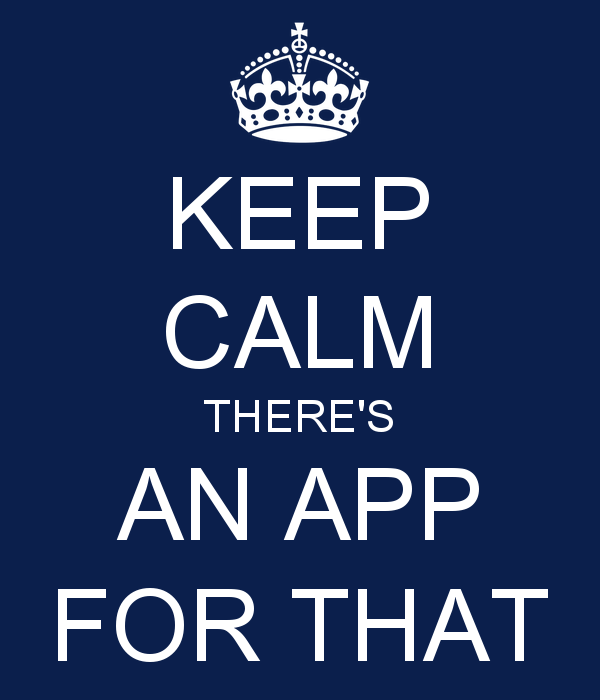# There's an App for that - and we have it!

Cotton Patch now has an app!

Why would you want the app?
No need for Facebook - you can watch our FB Live and shop right on the app!
Easy to navigate - there's even a search button!
Shop anytime you like!
App-only sales you won't want to miss!

 table div table+table+table+table+table+table+table+table+table+table+table+table+table+table+table+table+table+table+table+table+table+table+table+table+table+table+table+table+table+table+table+table+table+table+table div table{width:100%;padding:0}table div table+table+table+table+table+table+table+table+table+table+table+table+table+table+table+table+table+table+table+table+table+table+table+table+table+table+table+table+table+table+table+table+table+table+table div table img{width:96.23%;padding:0;float:none}table div table+table+table+table+table+table+table+table+table+table+table+table+table+table+table+table+table+table+table+table+table+table+table+table+table+table+table+table+table+table+table+table+table+table+table div table td{width:100%;padding:0 1.88% 18px}/* styles */# Meet me in the Club House!

Cotton Patch Club House is a new FaceBook group and we want you to be a part of it!
You will find:
An online community for friends of Cotton Patch Quilt Shop!
A place to chat and share photos of your projects.
A place to ask quilty questions, share tips and demos
A place for you to find sneak peeks and early access to items on our FaceBook Live Sunday Soiree!

 table div table+table+table+table+table+table+table+table+table+table+table+table+table+table+table+table+table+table+table+table+table+table+table+table+table+table+table+table+table+table+table+table+table+table+table+table+table+table div table{width:100%;padding:0}table div table+table+table+table+table+table+table+table+table+table+table+table+table+table+table+table+table+table+table+table+table+table+table+table+table+table+table+table+table+table+table+table+table+table+table+table+table+table div table img{width:96.23%;padding:0;float:none}table div table+table+table+table+table+table+table+table+table+table+table+table+table+table+table+table+table+table+table+table+table+table+table+table+table+table+table+table+table+table+table+table+table+table+table+table+table+table div table td{width:100%;padding:0 1.88% 18px}/* styles */# Instore Shopping is Back!

## We are open on Fridays and Saturdays from 10am to 3pm for instore shopping!

Masks are not required at this time, but are highly suggested.
We are excited to have you back in the shop and look forward to your visit!

 table div table+table+table+table+table+table+table+table+table+table+table+table+table+table+table+table+table+table+table+table+table+table+table+table+table+table+table+table+table+table+table+table+table+table+table+table+table+table+table+table div table{width:100%;padding:0}table div table+table+table+table+table+table+table+table+table+table+table+table+table+table+table+table+table+table+table+table+table+table+table+table+table+table+table+table+table+table+table+table+table+table+table+table+table+table+table+table div table img{width:96.23%;padding:0;float:none}table div table+table+table+table+table+table+table+table+table+table+table+table+table+table+table+table+table+table+table+table+table+table+table+table+table+table+table+table+table+table+table+table+table+table+table+table+table+table+table+table div table td{width:100%;padding:0 1.88% 18px}/* styles *//* styles */ Oh mom, I'm so comfy here on dad's pillow, do we have to go to work today? Yes, Freddie, we do.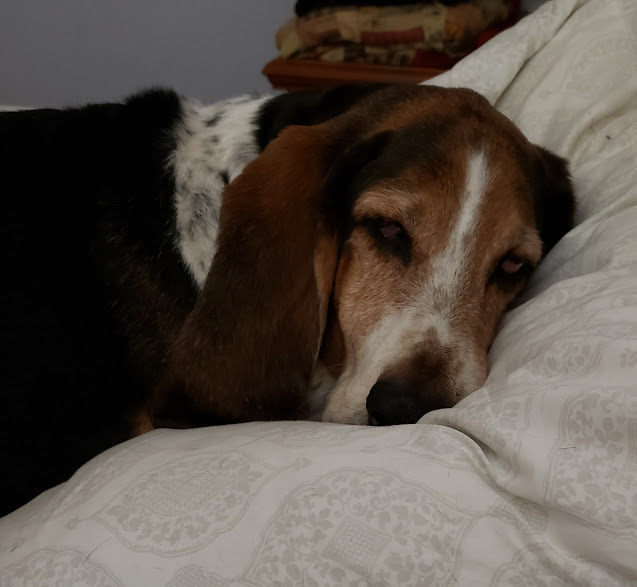Like   Pin   +1